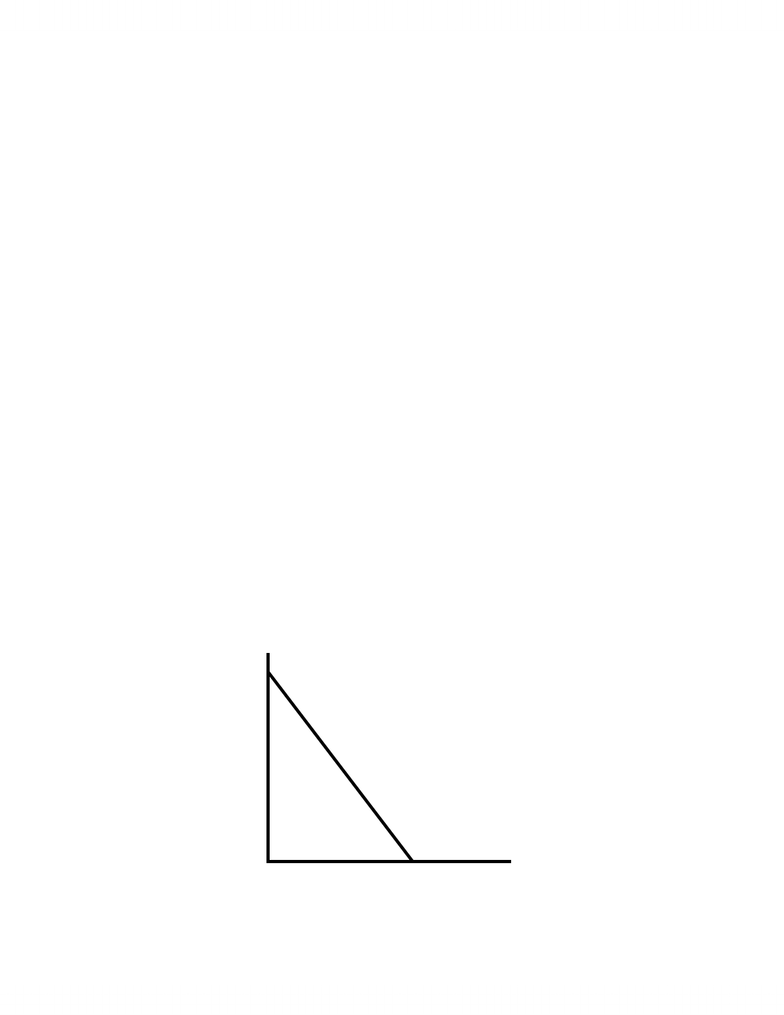Class Notes (1,100,000)
CA (630,000)
U of C (8,000)
BSEN (50)
Lecture

BSEN 401 Lecture Notes - One Unit, Opportunity Cost, Lincoln Near-Earth Asteroid Research

Department
Course Code
BSEN 401
Professor
William Huddleston

This preview shows page 1. to view the full 5 pages of the document..
LINEAR PRODUCTION POSSIBILITIES CURVES
We can express a Production Possibilities Curve with constant Opportunity Cost as a linear
equation.
e.g. Suppose that the equation for production of Y and X is Y = 150 – 2X where Y is the
quantity of Y and X is the quantity of X.
We will graph this with Y on the vertical axis and X on the horizontal axis (as usual).
i) What is the maximum output of Y if no X is produced, i.e., X = 0?
X = 0, => Y = 150 – 2(0) = 150
This gives the ‘vertical intercept’ of the diagram, i.e., the amount of Y when X = 0.
ii) What is the maximum output of X if no Y is produced (Y = 0)?
Y = 0, => 0 = 150 – 2X => X = 150/2 = 75
This gives the ‘horizontal intercept’ of the diagram, i.e., the amount of X when Y = 0
These two pieces of information are sufficient to draw the Production Possibilities Curve
because the function is linear, i.e., a straight line between two points.
150
75
Quantity of Y
Quantity of X
iii) What is the slope of the Production Possibilities Curve?
- 1 -
You're Reading a Preview

Unlock to view full version

Only page 1 are available for preview. Some parts have been intentionally blurred.The slope of this line = rise/run = Y/X = -150/75 = -2. The coefficient of X in the linear
equation gives us the slope directly as –2 since an increase in X of 1 causes a decrease in Y of 2.
iv) What is the Opportunity Cost of an increase of one unit of X given full employment?
The opportunity cost of a one unit increase in X is 2 units of Y.
Opportunity Cost = - X/Y = -(-2) = 2
The Opportunity Cost is constant so the function is linear.
v) What is the Opportunity Cost of an increase of one unit of Y given full employment?
One unit of Y costs 75/150 = ½ unit of X.
We could also find this by expressing our equation in terms of X to get X = 75 – Y/2. This
would also show that opportunity cost of Y is X/2.
The simplest way to find the Opportunity Cost of X is to realize that Y/X = 1/(X/Y).
The opportunity cost of one unit of X is 1/-(X/Y) = ½.
vi) Suppose that the economy presently produces 40 units of X and 50 units of Y. What is the
opportunity cost of increasing X to 50 units? Of increasing X to 60 units?
The combination 40X and 100Y are below the production possibilities curve because
production of 50Y would allow production of 50X at full employment. An increase in the
production of X to 50 units would have 0 opportunity cost therefore.
Production of 60 units of X would allow production of a maximum of 30 units of Y. The
opportunity cost of moving from (50, 50) to (60, 30) is 20 units of Y.
Sources of Growth:
Growth of the output possibilities of an economy implies increased productive capacity.
This can occur in two ways.
1. An increase in resources
- 2 -
You're Reading a Preview

Unlock to view full version